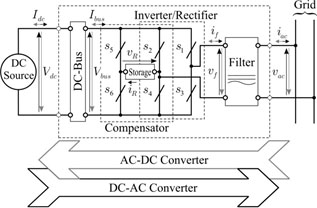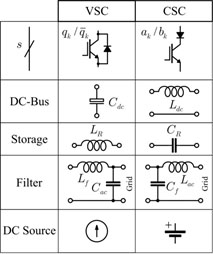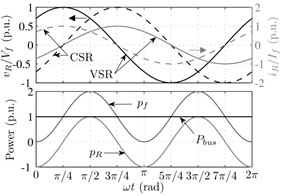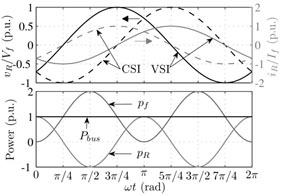LIBRARY

# Compensation of DC-Link Pulsation in Single-Phase Static ConvertersFig. 1. (a) Single-phase AC converter: Generalized converter with oscillating power compensation.
Single-phase AC power has a typical frequency that corresponds to twice the electrical grid frequency. Processing of such power made by voltage source converters (VSC) or current source converters (CSC) means there is a low-frequency oscillation of current or voltage along the DC link in the VSC or CSC, respectively. In order to avoid interference from these oscillations during power processing, it is necessary to use a large capacitor or inductor as part of the VSC or CSC. These oscillations degenerate the quality of power processing and are present in both operation modes: rectifier (AC-DC converter) and inverter (DC-AC converter).

In terms of hardware, compensation of such oscillating power can be achieved by adding an auxiliary leg and a energy storage circuit element to a standard converter. Here, these constitute the oscillating power compensator. The energy storage element is responsible for absorbing and delivering the oscillating power, which is naturally required by a single-phase converter. A general (VSC/CSC) single-phase converter, with power compensator, is presented in Fig. 1(a). Fig. 1(b) illustrates the particular composition for a VSC and CSC, with oscillating power compensation included.

Fig. 2(a) shows AC voltage and current waveforms related to single-phase (VSC/CSC) converters. In Figs. 2(b) and 2(c) are shown the waveforms for the power compensator working as a rectifier and as an inverter, respectively. In order to achieve an effective compensation, the power compensator needs to generate a voltage, as illustrated in Figs. 2(b) and 2(c) (top). In Figs. 2(b) and 2(c) (bottom) is shown the behavior of the power on the AC side, DC bus and the compensator. It is possible to see that the power oscillation does not spread across the converter, so the power in the DC bus is continuous.Fig. 1. (b) Legend for VSC and CSCFig. 2. (a) Waveforms of the converters with oscillating power compensation, where (b) and (c) (top) are in the compensator side and (bottom) are the power in the converters: Grid side.Fig. 2. (b) Working as rectifier.Fig. 2. (c) Working as inverter.